Next:5.2.2 Red-Black Trees: InsertionsUp:5.2 Red-Black TreesPrevious:5.2 Red-Black Trees

## 5.2.1 Height of a Red-Black Tree

Result 1

In a RBT, no path from a node x to a leaf is more than twice as long as any other path from x to a leaf.

Let bh(x) be the black height of x. Then the length of a longest path from x to a leaf

= 2bh(x)  {a path on which red and black nodes alternate}

Length of the shortest possible path from x to a leaf

bh(x)  {a path that only contains blacks}

Hence the result.

Result 2

A red-black tree with n internal nodes has height at most

2log(n + 1)

Consider any node x and the subtree rooted at x. We will first show that this subtree has at least

2bh(x) - 1  internal nodes
We do this by induction on the height of x. If h(x) = 0, bh(x) = 0, x is leaf and hence the subtree has no internal nodes, as corroborated by

20 - 1 = 0

Let h(x) > 0 and let x1 and x2 be its two children (Figure 5.11)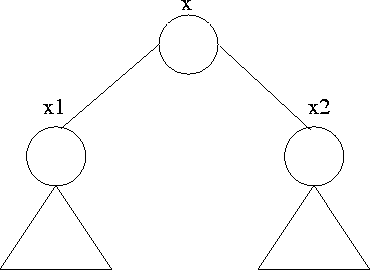Note that h(x1), h(x2) are both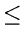h(x) - 1. Assume the result to be true for x1 and x2. We shall show the result is true for x.

Now,

 bh(x1)bh(x)  and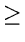bh(x) - 1 bh(x2)bh(x)  andbh(x) - 1

Therefore, the tree with root x1 has at least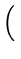2bh(x) - 1 - 1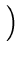internal nodes
whereas the tree with root x2 has at least2bh(x) - 1 - 1internal nodes
Thus the tree with root x has at least

1 + 2bh(x) - 1 - 1 + 2bh(x) - 1 - 1 =2bh(x) - 1&nbsp;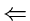internal nodes
To complete the proof, let h be the height of the tree. Then

bh (root)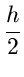Thus

 n/TD>2h/2 - 1h/TD>2log(n + 1)Next:5.2.2 Red-Black Trees: InsertionsUp:5.2 Red-Black TreesPrevious:5.2 Red-Black Trees
eEL,CSA_Dept,IISc,Bangalore